This video was brought to you by GeneralPAC.com, making power systems Intuitive, Open and Free for Everyone, Everywhere. Consider subscribing and supporting through patreon.com/GeneralPAC. This is a mechanism for you to support us financially so we can continue making high quality power system video tutorials. Our corporate sponsor for this topic is AllumiaX.com from Seattle, Washington. Contact them for industrial and commercial power system studies.

Fault Analysis in Power Systems Part 3b. This is the second video in Part 3 of the series on fault analysis in power system where we will hand calculate the current and voltage quantities for various types of fault.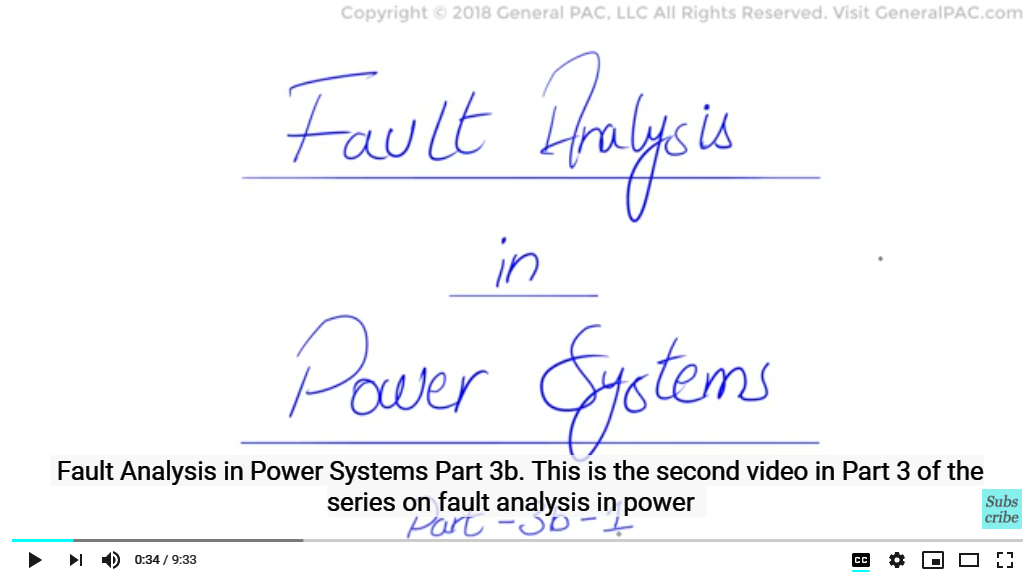In this video we will see how phase currents and voltages are calculated for a single line to ground fault on line A of the 13.8kV system. So Let’s get started with our previous example

We have a three phase synchronous generator having a short circuit capability of 600 MVAsc connected to a 115 kV bus. A delta – Wye transformer rated at 30 MVA connects the 115 kV bus to the 13.8 kV bus and has an impedance of 10 % at 30 MVA. Step 1 of our calculation asks us to convert our system into per unit values and that is exactly what we did in part 2b of our series. Here we draw the same network again.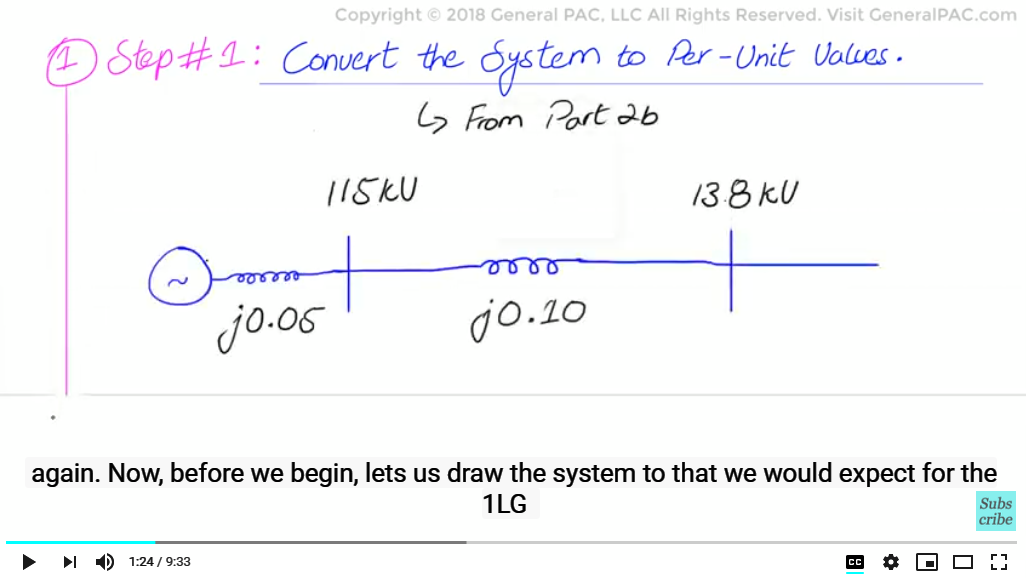Now, before we begin, lets us draw the system to that we would expect for the 1LG fault on the 13.8kV bus with the diagram below.

Now, when the fault occurs at the point of the fault, we see that the fault current goes to ground, then it returns from the grounded system on the wye side of the transformer neutral and makes a circulating loop.

We also see that the fault current coming from the 115kV side of the system which is the source. We note that fault current is not present on phase b and c lines on the 13.8kV system and because we have a Delta-AB Wye-grounded transformer connection, fault current is only present on Phase A & C side of the transformer but not Phase B.

Now, let us go through the calculations by hand and prove this. Next we move to step 2, which asks us to identify the type of fault involved.

Now, as mentioned before, we have a single line to ground fault which is an unsymmetrical fault (meaning an unbalanced fault type). This type of fault produces all sequence quantities, positive, negative and zero sequence components. We talk about current and voltage quantities. Next, we move to step no 3, which asks us to make an unfaulted sequence networks from the per unit converted system in Step 1. These are the individual sequence network diagrams which were covered in part 2c of the series, here we will redraw the same networks, so these are the unfaulted sequence network.

This is the positive sequence network diagram which is indicated by a voltage source.The negative sequence network diagram is very similar to the positive sequence network, however it does not have a voltage source.And the zero sequence network diagram also does not have the voltage source, however, it does represent the Delta wye transformer with a high voltage side open and low voltage side grounded.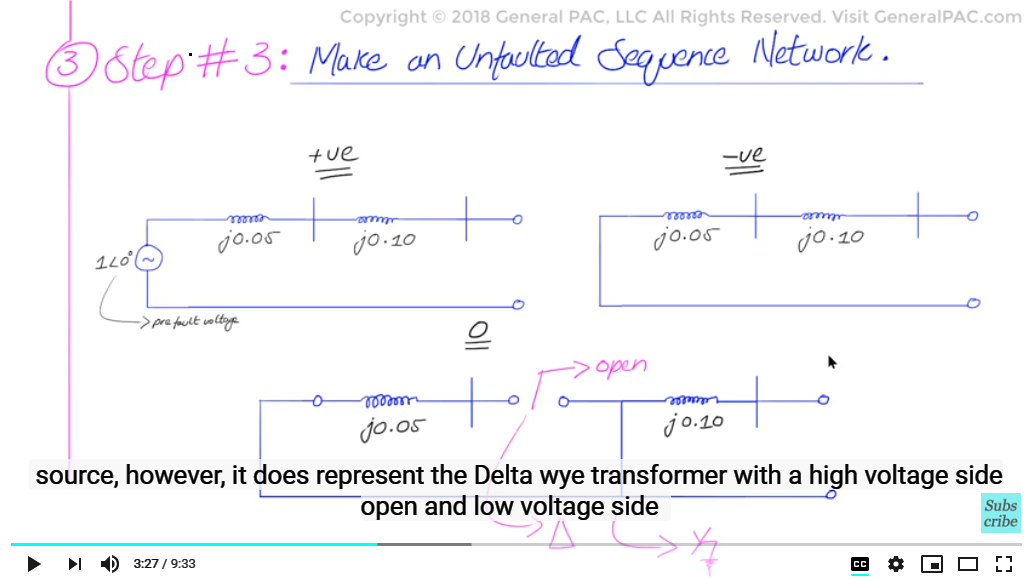And then, in Step no 4, we will draw the faulted sequence network, given that the fault is a single line to ground fault we will connect all three sequence networks in series. This we have explained in great detail in a separate video in Topic 2: Derivations of sequence network diagrams of our series. Here we just redraw the series connected faulted networks.

Let us describe this faulted sequence network diagram briefly. The top most sequence is the positive sequence network which can be easily identified by the voltage source. The middle is the negative sequence network which is very similar to the positive sequence but does not have a voltage source.

The bottom network is the zero sequence network which also does not have any voltage source. However, we do account for the transformer in the zero sequence network diagram (the open-circuit on the High Voltage side and short-circuit on the Low Voltage side).

Now let’s move towards step no 5, here we will hand calculate the sequence network quantities. Since we have an unbalanced network we will calculate all the three sequence components, positive, negative and zero sequence.

In this faulted sequence network, we will see that all impedances are connected in series in a single loop and there is only one voltage source, which is 1pu angle 0 degrees in this entire loop (this comes from the positive sequence).

Therefore, it can be deduced that all three sequence components current values and current quantities, which is positive, negative, zero sequence currents will be equal and they can be calculated by dividing the voltage with the sum of the impedance for the entire loop. The sum of the impedance of the entire loop will now be j0.05 + j0.10 p.u. This is the positive sequence impedance plus j0.05 + j.10 p.u. This is for the negative sequence impedance and finally plus j0.10 only for the zero sequence impedance.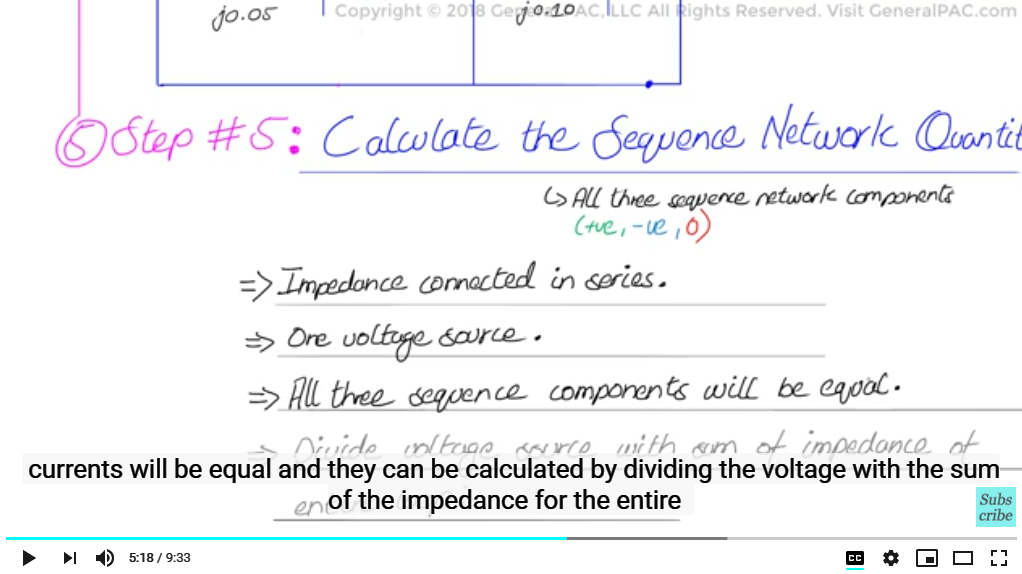Now remember, if there is a zero sequence impedance, there is open-circuit for the Zero Sequence network so we cannot consider the j.05 pu generator impedance).

So the total impedance of the entire loop will be j0.40 per unit.Positive sequence current in this loop is equal to the Prefault voltage which is

I_fa^((1) )=(prefault voltage source)/(total impedance)=(1∠0° pu)/(j0.40 pu)=-j2.5pu

1 angle 0 degree divided by j0.40 p.u and that gives us negative –j2.5 per unit. Now this is the positive sequence current quantity. Since this is a per unit value we will need to find the base of the current and multiply the per unit value with base value to find the actual current quantity in amperes.

I_Base=30MVA/(√3*13.8kV)=1255A

The base value of current is equal to 30 MVA divided by square root of 3 times 13.8 KV which results to 1255 Amps Multiply that with –j2.5 and we get the actual ampere quantity of the current.

I_fa^((1) )=-j2.5pu*1255A=3138A∠"-" 90°

Which equals to 3138 Amperes at the angle -90 degrees.

As we stated earlier that all sequence component values are equal because the positive, negative, and zero sequence networks are connected in series. So the same current would flow though all three sequence networks which means that the positive sequence current is equal to the negative sequence current which equals to zero sequence current which equals 3138 Amps at the angle of -90 degrees.

I_fa^((1) )=I_fa^((2) )=I_fa^((0) )=3138 Amps angle-90 degree

As a final step no 6, we have to convert these sequence component values into phase current values by plugging in sequence components values and a operators in these three familiar equations below:

I_(∅a)=I_fa^((1))+I_fb^((2))+I_fc^((0))
I_(∅b)=a^2∙I_fa^((1))+a∙I_fa^((2))+I_fa^((0))
I_(∅c)=a∙I_fa^((1))+a^2∙I_fa^((2))+I_fa^((0))
I_(∅a)=(3138∠"-" 90°)+(3138∠"-" 90°)+(3138∠"-" 90°)= 9413A ∠"-" 90°
I_(∅b)=(1∠240°)(3138∠"-" 90°)+(1∠120°)(3138∠"-" 90°)+(3138∠"-" 90°)=0
I_(∅c)=(1∠120°)(3138∠"-" 90°)+(1∠240°)(3138∠"-" 90°)+(3138∠"-" 90°)=0

What we will find is that;

For line a current on 13.8kV side, we get 9413 Amps at the angle of -90 degrees.

For Phase b line current on the 13.8kV side, we get 0, the math just happens to be that we get 0 amperes.

And for Phase c line current on the 13.8kV side, we also get 0.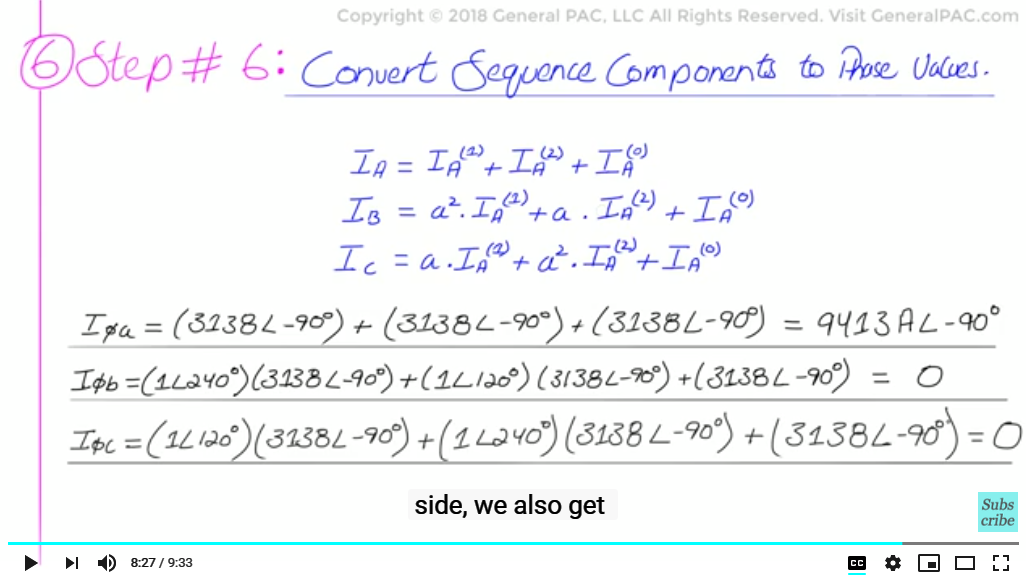This is exactly the same as what we assumed in our video on single line to ground fault derivations and intuitively only the phase shorted to ground is supposed to carry the short circuit current, in this case the faulted phase is phase A which has 9413 Amps at -90 degrees. The remaining phases (meaning Phase B and Phase C) have no fault current flowing, therefore they are zero.

So, now in the next part, what we will do is, we actually calculate the voltage quantities on the 13.8 Kv side, so this will be split into the second part, Part 3b.

We hope, that you find this tutorial valuable and useful as a professional or a student. Please support us by becoming our patron and donating through patreon.com/generalpac. Your generous donation will help us continue making high quality power system video tutorials. Thank you.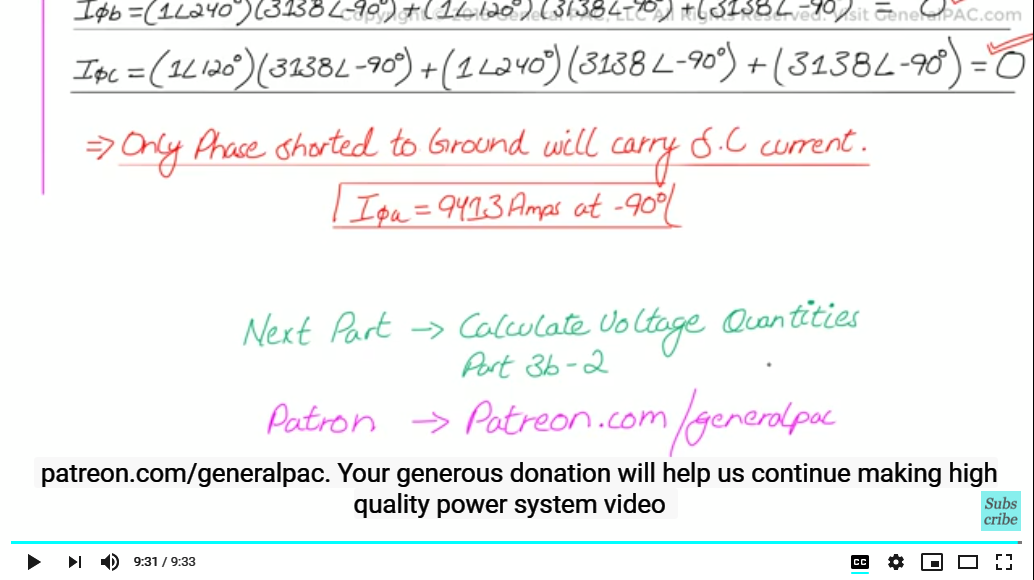#### Greetings from the GeneralPAC Team!

We make high-quality Power Systems Video Tutorials on complex topics that are free and open to everyone!  Thank you so much for supporting us through Patreon so can continue doing good and valuable work.

What is Patreon and why do we use it?

Patreon is a fantastic portal that allows our fans and community to make monthly contribution (like Netflix subscription) so we can continue creating high-quality power systems video tutorials. In return, you get access to incredible perks like voting on future topics, getting your questions answered, access to VIP Q/A webinars with the creators of GeneralPAC, and much more! We THANK YOU for supporting us

Why do we need your support?

An incredible amount of time and effort is needed to develop high-quality video tutorials. Each video (Part 1 for example) takes approximately 10 hours to complete which includes learning the concept ourselves, brainstorming creative ways to teach and explain the concepts, writing the script, audio recording, video recording, and editing. It's no wonder why Hundreds-of-Thousands of people have watched, liked, subscribed, and left positive comments on Youtube channel. Your support truly makes all the difference.